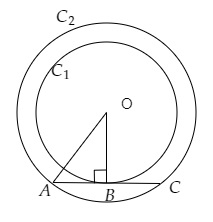# Out of the two concentric circles, the radius of the outer circle is 5 cm and the chord AC of length 8 cm is a tangent to the inner circle. Find the radius of the inner circle.

Given:

Out of the two concentric circles, the radius of the outer circle is 5 cm and the chord AC of length 8 cm is a tangent to the inner circle.

To do:

We have to find the radius of the inner circle.

Solution:Let $C_1$ and $C_2$ be the two circles having the same centre $O$. AC is the chord that touches $C_1$ at point $B$.

Join $OB$.

$OB\ perp\ AC$

We know that,

Perpendicular from the center of a circle to a chord bisects the chord.

This implies,

$AB = BC = \frac{8}{2}=4\ cm$

In right angled triangle $AOB$,

By Pythagoras theorem,

$OA^2 = AB^2 + OB^2$

$OB^2 = 5^2 - 4^2$

$= 25 - 16$

$= 9$

Therefore,

$OB = 3\ cm$

The radius of the inner circle is $3\ cm$.

Updated on: 10-Oct-2022

37 Views